Courses
Courses for Kids
Free study material
Free LIVE classes
More

# Determination of Income and Employment Class 12 Notes CBSE Macro Economics Chapter 4 [Free PDF Download]## Revision Notes for CBSE Class 12 Macro Economics Chapter 4 - Free PDF Download

In this chapter, we study the determination of National Income under the assumption of a fixed price of final goods and a constant rate of interest in the economy.

### Aggregate Demand and its Components

Aggregate Demand refers to the total demand for final goods and services in an economy during a year. It is denoted by AD.

The Components of Aggregate Demand Are

1. Private consumption demand $(C)$

2. Private investment demand $(I)$

3. Purchase of goods and services by the government $(G)$

4. Demand for net exports $(X – M)$

Thus, $\text{AD = C + I + G + NE}$ (sum of four components: consumption, investment, government purchase and net export)

Aggregate Demand is very important for economists as it provides the tool to measure the strength of an economy. Usually, economists evaluate the total market for things produced in an economy for a year. So, if the aggregate demand is high then the economy is strong, meaning it can sell many products.

Last updated date: 03rd Jun 2023
Total views: 531k
Views today: 13.15k

### Related Chapters

More Free Study Material for Determination of Income and EmploymentNcert solutionsImportant questions

## Access Class 12 MacroeconomicsEconomics Chapter 4 – Determination of Income and Employment Notes

• It refers to the total value of all final goods and services that are planned to be purchased by all sectors of the economy at a given level of income over a given time.

• AD denotes the total expenditure on goods and services in an economy over a given time.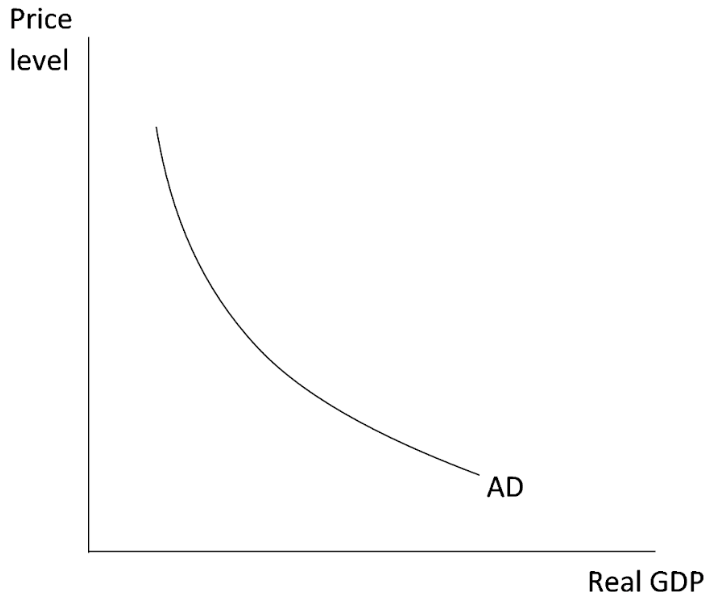Components of Aggregate Demand in an Open Economy:

• Consumption expenditure by households (C).

• Investment expenditure (I).

• Government consumption expenditure (G).

• Net exports (X – M).

Therefore,

AD = C + I + G + (X – M)

Components of Aggregate Demand in Closed Economy:

• A three sector economy;

AD = C + I + G

• A Two sector economy;

Ex-ante aggregate demand:

• The term ex-ante refers to what has already been planned.

• So, it is planned aggregate demand.

Ex-post aggregate demand:

• Actual consumer spending and business capital investment are included in ex-post aggregate demand.

• In other words, the ex-post describes what actually occurred.

### Aggregate Supply (as):

• It is the monetary value of all final goods and services purchasable by an economy over a specific period.

• It refers to the movement of goods and services in the economy.

• AS is nothing more than national income because the money value of final goods and services equals net value-added.

AS = C + S

The aggregate supply represents the country's national income.

AS = Y (National Income)

### Consumption Function:

• Household income is the most important determinant of consumption demand.

• The relationship between consumption and income is described by a consumption function.

• The most basic consumption function assumes that consumption changes at the same rate as income.

Equation of Consumption Function

$C= {\overline C} + cY$

C = Consumption

${\overline C}$ = Autonomous consumption

cY= Induced consumption

Y= Income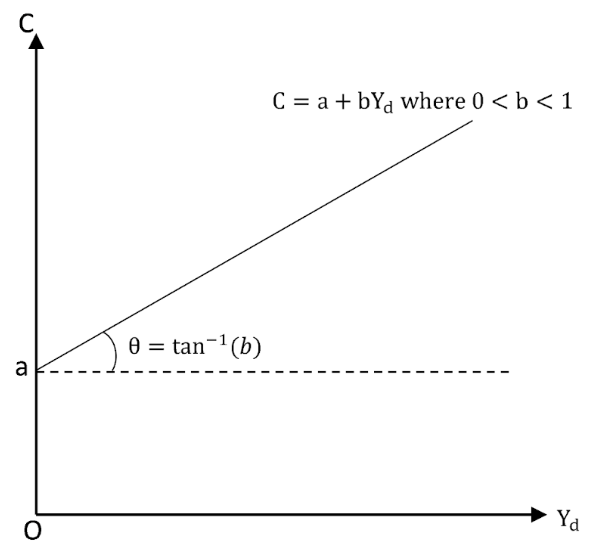The consumption curve starts from the Y axis because, even when the income is zero, there is some consumption.

Autonomous Consumption:

• Autonomous consumption is denoted by ${\overline C}$ and represents consumption that is unaffected by income.

• When consumption occurs even when income is zero, it is due to autonomous consumption.

• Hence this consumption is independent of income.

Induced Consumption:

• The induced component of consumption, cY, demonstrates consumption's dependence based on earnings/ income.

• Hence, this consumption is dependent on income.

### Propensity to Consume

Propensity to consume is of two types:

a. Average Propensity to Consume (APC)

• It refers to consumption per unit of income.

• It is denoted as $\frac{\mathrm C}{\mathrm Y}$

• APC>1: APC is greater than one if consumption exceeds national income before the break-even point i.e., APC > 1.

• APC=1: When APC=1, consumption equals national income at the break-even point.

• APC<1: When APC is less than one after the break-even point consumption exceeds national income.

• Inverse Relation with Income: APC decreases as income increases, hence income and APC are inversely related.

• APC can never be zero: APC can never be zero because autonomous consumption exists even at a zero level of national income.

b. Marginal Propensity to Consume (MPC)

• It is the change in income per unit change in consumption.

• It is represented by c and equals $\frac{\mathrm{ΔC}}{\mathrm{ΔY}}$ , where $\triangle\mathrm C$ is change in consumption and $\triangle\mathrm Y$ is the change in income.

• That is MPC= $\frac{\mathrm{ΔC}}{\mathrm{ΔY}}$

• MPC=1: If all the extra income is consumed, then $\triangle\mathrm C=\triangle\mathrm Y$, resulting in MPC= 1.

• MPC = 0: However, if the entire additional income is saved, $\triangle\mathrm C$=0 , and MPC= 0.

• Constant MPC: MPC is the slope of the consumption curve, and it remains constant in the short run.

• APC value > MPC.

### Saving Function:

• The functional relationship between saving and national income is referred to as the saving function.

Equation of saving function

S = f (y)

Where,

S = Saving

Y = National Income

f = Functional relationship.

$S\text{ }=\text{ }-a\text{ }+\text{ }\left( 1\text{ }-\text{ }b \right)\text{ }y$

Here,

1-b = MPS

Y= Income

-a = Savings, when Y is 0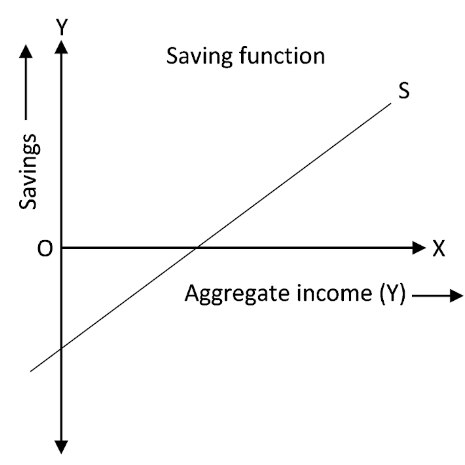In the diagram, the S curve starts from below 0, as when income is zero, the savings are negative.

### Propensity to Save

Propensity to Save is of two types:

a. Average Propensity to Save (APS)

• It refers to the savings per unit of income.

• It is denoted as $\frac{\mathrm S}{\mathrm Y}$

• Savings can never be equal to or greater than income, so APS can never be one or more than one.

• At the break-even point, when C= Y,  APS can be zero, as here S= 0. This is because when a person consumes equals to what he/she earns, there are no savings.

• When consumption exceeds income at income levels lower than the break-even point, APS can be negative.

• With an increase in income, APS rises. Hence, APS and income are directly related.

b. Marginal Propensity to Save (MPS)

• It is the change in savings per unit of income change.

• It is represented by s and equals 1-c. This is because 1 is the whole, and if we less consumption from it, we can get the savings.

• It follows that, S + C = 1, i.e, the total of savings and consumption equals one.

• That is MPS= $\frac{\mathrm{ΔS}}{\mathrm{ΔY}}$

• MPS ranges from 0 to 1.

• MPS is the saving curve's slope.

• In the short run, MPS remains constant.

Relation between APC and APS

The product of APC and APS equals one.

It can be demonstrated as follows:

APC + APS = 1.

Y = C + S

Dividing both side by Y, we get

$\dfrac{Y}{Y}= \dfrac{C}{Y} + \dfrac{S}{Y}$

That is,

1= APC + APS

As, $\mathrm{APC}=\frac{\mathrm C}{\mathrm Y},\;\mathrm{APS}=\frac{\mathrm S}{\mathrm Y}$

Therefore,

APC + APS = 1

Relation between MPC and MPS

We know MPC + MPS = 1

Also,

Y = C +S

Hence

$\Delta Y = {\Delta C} + {\Delta S}$ - (i)

Where,

$\Delta C=$ Change in consumption

$\Delta Y=$ Change in income

$\Delta S=$ Change in savings

And,

$\mathrm{MPC}=\dfrac{\Delta C}{\Delta Y}$

And,

$\mathrm{MPS}=\dfrac{\Delta S}{\Delta Y}$

So dividing eq (i) with change in Y on both sides

$\dfrac{\Delta Y} {\Delta Y} =\frac{\Delta C}{\Delta Y} + \frac{\Delta(Y-C)}{\Delta Y}$

$1=\frac{\Delta C}{\Delta Y}+\frac{\Delta S}{\Delta Y}$

1 = MPC + MPS

### Investment:

• An investment is an asset or item purchased with the intention of earning income or increasing in value. An increase in the value of an asset over time is referred to as appreciation.

• Induced Investment: It is defined as an investment that is based on profit expectations and is directly influenced by income level.

• Autonomous Investment: It is defined as an investment that is not affected by changes in income and is not motivated solely by a profit motive.

Ex-ante Investment:

• Ex-ante investment refers to the investment made by firms in the economy during a specific period. The planning is done with future expectations in mind.

Ex-post Investment:

• This refers to the actual investment made by all entrepreneurs in the economy during a given period. It is the outcome of actual investment.

### Equilibrium Level of Income

• The equilibrium level of income is only determined when AD = AS or S = I, i.e., when the flow of goods and services in the economy equals the demand for goods and services.

• However, it cannot always be at full employment and may be less than full employment.

### Short Run Equilibrium Output:

The quantity of real GDP that will exist when AD intersects Short Run Aggregate Supply in a short-run macroeconomic equilibrium is the amount of aggregate output produced.

Assumptions:

• Closed Economy: In the framework of a two-sector model (households and firms), the determination of equilibrium output will be investigated. It implies that there is no government or international sector. Such that AD=C+I

• Self contained Investment: It is assumed that investment expenditure is self-contained, i.e., investments are unaffected by income levels.

• Short-period analysis: This analysis is with reference to short period only.

• The level of output where the Aggregate Demand equals Aggregate Supply (AD = AS) in an economy.

• It indicates that whatever the producers intended to manufacture during the year is exactly equal to what the buyers intended to purchase during the year.

Here,

AD = C + I (for a two-sector economy), and

AS = C + S

That is,

AS = Aggregate Supply,

C = Consumption,

I = Investment,

S = Saving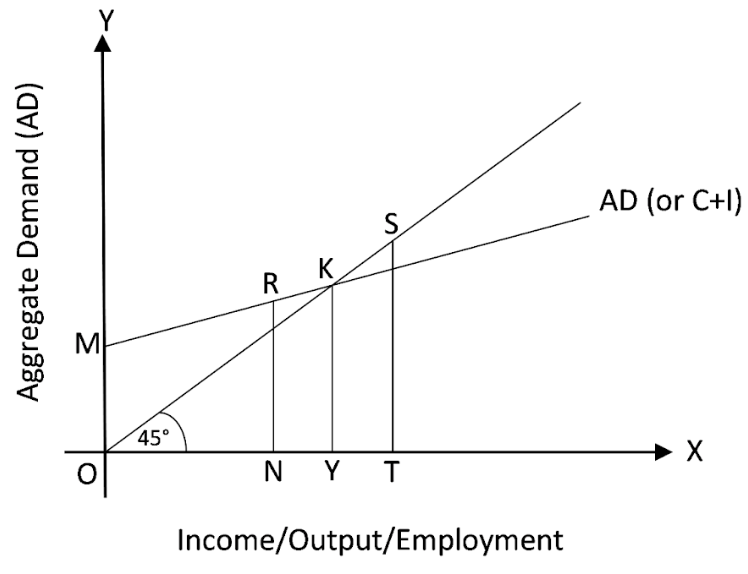The diagram represents aggregate demand, and the situation of equilibrium at point K, where AD=AS, and the level of equilibrium output at point Y.

### Two different Situations:

• AD > AS: In this case aggregate demand exceeds aggregate supply, and a situation of unfulfilled demand persists. To curb this situation, the producers will enhance the level of output and production such that AS could increase and become equal to AD, and the situation of equilibrium is restored. This is shown as point R in the diagram, where AD>AS.

• AD < AS: In this case aggregate demand is less than the aggregate supply, and a situation of unwanted stocks persists. To curb this situation, the producers will decrease the level of output and production such that AS could decrease and become equal to AD, and the situation of equilibrium is restored. This is shown as point S in the diagram, where AD<AS.

### Savings-Investment Approach:

• It is the point of equilibrium, where S=I. Here, S = savings, or withdrawal, and I = investment, or injection.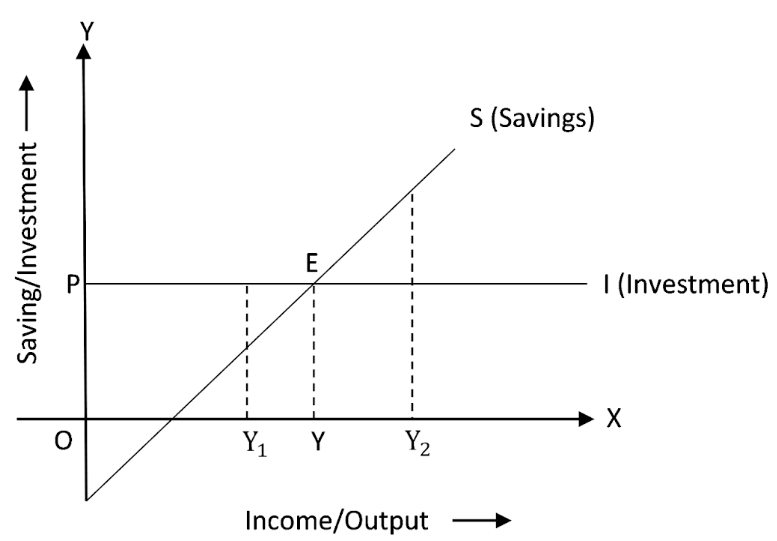Explanation of Diagram

• Point E on the diagram represents the equilibrium point where S = I. The quantity of money removed (S) from the economy equals the amount of money put (I) into the economy at this point.

• In the economy, AD = AS at this level.

Two Situations:

• S > I: At this point, some of the anticipated output remains unsold, forcing companies to keep unsold items on hand. In order to clear the stocks, producers will cut production, resulting in a decrease in output. As a result, the economy's income decreases. Less income means less savings, and this cycle will continue until saving equals investment.

• S > I: People spend more money than is necessary to purchase the projected output when S > I. This means that AD outnumbers AS in the economy.  As a result, manufacturers will increase output to compensate for the situation. As a result, investment rises to the point where it equals investment.

### Equilibrium

So, equilibrium is reached when:

We already know that AD is the sum of Consumption (C) and Investment (I):

AD = C + I... (ii)

Additionally, AS is the sum of consumption (C) and saving (S):

AS = C + S... (iii)

When we substitute (ii) and (iii) into (i), we get:

C + S = C + I, or

S = I

Note: It's important to remember that AD, AS, Savings, and Investment are all ex-ante variables.

### Types of Employment

• Full employment: This occurs when all those who are able and willing to work at the prevailing wage rate are given the opportunity to do so.

• Voluntary unemployment: This occurs when a person is able to work but unwilling to work at the prevailing wage rate.

• Involuntary unemployment: This occurs when a worker is able and willing to work at the prevailing wage rate but is unable to find work.

• Under employment: It occurs when all those who can work at current wage rates are unable to find work. It refers to the economic situation in which AS= AD or S = I, but there is insufficient labour force utilisation.

### Multiplier Mechanism:

• The multiplier shows us what the eventual change in income will be as a result of a change in investment. Changes in investment lead to changes in income.

• The aggregate demand rises when the autonomous measures (A) rise.

• As a result, output and income will rise in the next round, causing consumption and the AD to rise. This is referred to as the multiplier mechanism.

• It is represented symbolically by:

$\Delta I\text{ }\to \text{ }\Delta Y\text{ }\to \text{ }\Delta C\text{ }\to \text{ }\Delta Y$

The operation of a multiplier can be illustrated using the table below, which is based on consumption, that is, $\Delta K = 1000$ and $MPC = \dfrac{4}{5}.$

### Working of  multiplier:

The process of income generation is shown below.

 Rounds $\Delta I$ $\Delta Y$ $\Delta C$ 1 1000 1000 $\dfrac{4}{5}\text{ }\times \text{ 1000 = 800}$ 2 - 800 $\dfrac{4}{5}\text{ }\times \text{ 800 = 640}$ 3 - 640 $\dfrac{4}{5}\text{ }\times \text{ 640 = 512}$ 4 - 512 $\dfrac{4}{5}\text{ }\times \text{ 512 = 409}\text{.6}$ $\downarrow \infty$ $\downarrow \infty$ $\downarrow \infty$ $\downarrow \infty$ Total 5000

According to the above table, as $\text{MPC = }\dfrac{4}{5},$ the initial increase in investment of Rs 1000 results in a total increase in income of Rs 5000. From the whole increase in income, Rs. 4000 will be spent and Rs. 5000 will be saved.

The derivation of the sum of total increase in income is shown below.

$=1000+\dfrac{4}{5} \times 1000\left(\dfrac{4}{5}\right)^{2} \times 1000\left(\dfrac{4}{5}\right)^{3} \times 1000+\ldots \ldots \ldots \infty$

$=1000\left[1+\dfrac{4}{5}+\left(\dfrac{4}{5}\right)^{2}+\left(\dfrac{4}{5}\right)^{3}+\ldots \ldots . \infty\right]$

$=1000 [\dfrac{1} {1} - \dfrac {4} {5}]$

$=1000 \times \dfrac {5} {1}$

$=\text { Rs. } 5000 \text { crores. }$

### Investment Multiplier:

• The investment multiplier (K) is the ratio of the change in income (Y) caused by the change in investment (I).

• The value of the investment multiplier ranges from one to infinity.

$\mathrm K=\frac{\triangle\mathrm Y}{\triangle\mathrm I}\;\;\mathrm{or}\;\mathrm K=\;\frac1{1-\mathrm{MPC}}\;\mathrm{or}\;\mathrm K=\;\frac1{\mathrm{MPS}}$

### Excess Demand:

• It occurs when aggregate demand exceeds aggregate supply, resulting in full employment.

• Reasons for excess demand:

• Rise in household consumption demand due to increased propensity to consume.

• Rise in private investment demand because of higher provision and availability of credit facilities.

• Higher public (government) expenditure.

• Rise in demand for exports.

• Rise in supply of money

• Rise in disposable income.

• Impact of Excess demand on:

• General Price Level: General price level increases as when aggregate demand exceeds aggregate supply at a full employment level, there is a situation of inflation in the economy

• Output: It has no impact on output, as the economy is already at full employment level, thus no idle capacity exists. Hence, one cannot raise the output more than already.

• Employment:  No impact on employment level. The economy is already operating at full employment equilibrium.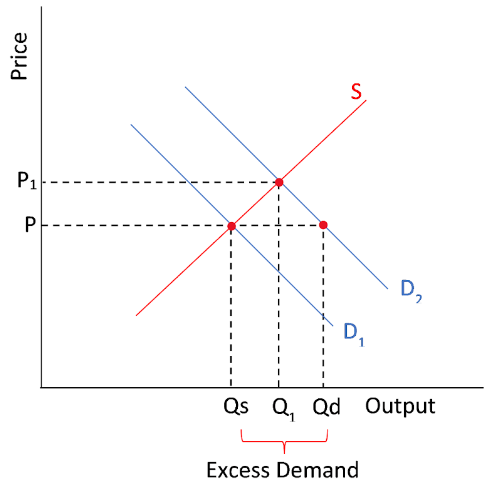### Deficient Demand:

• It occurs when AD falls short of AS at full employment. To put it another way, AD < AS is at full employment. It's referred to as deficient demand.

• Reasons for Deficient demand:

• Fall in household consumption demand due to decreased propensity to consume.

• Fall in private investment demand because of lesser provision and availability of credit facilities.

• Reduced public (government) expenditure.

• Fall in demand for exports.

• Fall in supply of money

• Fall in disposable income.

• Impact of Deficient demand on:

• General Price Level: General price level falls as when aggregate demand is less than aggregate supply at a full employment level, there is a situation of deflation in the economy

• Output:  Low output levels, due to unemployment, and reduced investment.

• Employment:  Low employment levels, as there will be a case of involuntary unemployment.

Diagram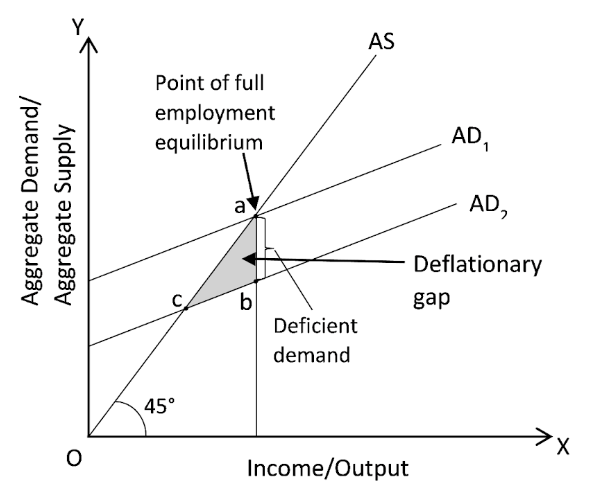### Inflationary Gap:

• The difference between actual aggregate demand and the level of aggregate demand required to achieve full employment is known as the inflation gap.

• It assesses the magnitude of excess demand.

• The area between FE represents the inflationary gap, as here aggregate supply, EM, is less than aggregate demand FM.

• As the output could not be increased beyond the full employment level, prices will rise, and there will be a situation of inflation in the economy.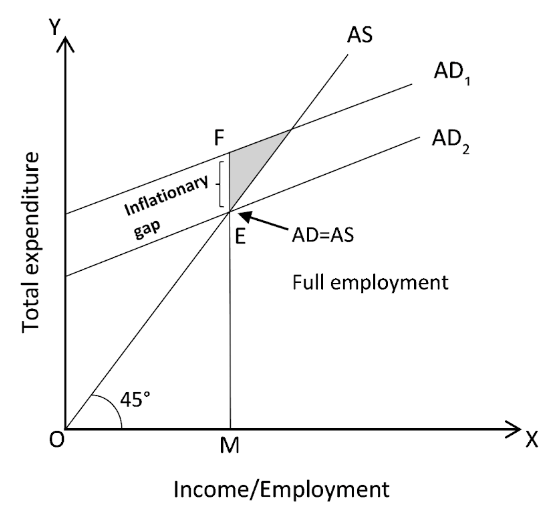### Deflationary Gap:

• Deflationary gap refers to the difference between the actual aggregate demand and the level of aggregate demand required to achieve full employment.

• It assesses the degree of deficient demand.

• The area between a and b shows deflationary gap, as here the Aggregate supply is greater than that of aggregate demand.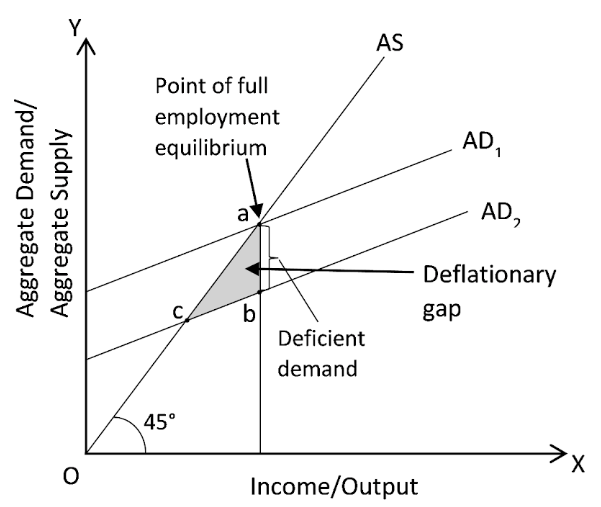### Mechanism to Control Excess Demand or Deficient Demand:

1. Fiscal Policy:

Fiscal policy refers to the general government's expenditure and income policies used to achieve its objectives. It includes:

1. Change in taxation:

Taxation is used to represent revenue policy.

• Excess Demand: During an inflationary period, the government raises taxes, resulting in a loss in people's purchasing power. This is due to the fact that in order to limit excess demand, the economy's liquidity must be reduced.

• Deficient Demand: In case of deficient demand, tax rates

1. Change in public expense:

The government must invest heavily in public works projects like roads, buildings, and irrigation systems.

• Excess Demand: During an inflationary period, the government should limit (lower) its expenditure on public works such as roads, buildings, and irrigation projects, therefore reducing people's money income and consumer requirements.

• Deficient Demand: During deficient demand, the government should increase its expenditure on public works such as roads, buildings, and irrigation projects, therefore increasing people's money income and consumer requirements.

1. A Shift in public borrowing:

• Excess Demand: This measure implies that the government should borrow money from the general population, which reduces people's purchasing power by leaving them with less money. As a result, during periods of high demand, the government should resort to increased public borrowing.

• Deficient Demand: This measure implies that the government should reduce the borrowings from the general population, which increased people's purchasing power. As a result, during periods of deficient demand, the government should resort to reduced public borrowing.

1. Monetary Policy:

It is the policy of a country's central bank to control the amount of money in circulation and the availability of credit in the economy.

1. Quantitative measures:

These are the monetary policy instruments that influence the overall supply of money/credit in the economy. These instruments do not direct or restrict credit flow to specific sectors of the economy.

1. Bank Rate

The bank rate is the interest rate at which a central bank lends money to commercial banks with no security.

• Excess Demand: Bank Rate should be increased in situations of excess demand, as due to this, the quantity of money accessible to banks decreases, and the commercial bank’s capacity to provide credit also falls. Hence the aggregate demand falls down with a low credit creation and supply of money in the economy.

• Deficient Demand: Bank Rate should be reduced in situations of deficient demand, as due to this, the quantity of money accessible to banks increases, and the commercial bank’s capacity to provide credit also rises. Hence the aggregate demand increases as a result of high credit creation and supply of money in the economy.

1. Cash Reserve Ratio (CRR)

It is the minimum percentage of a bank's total deposits that it must keep with the central bank. As a matter of law, commercial banks must keep a certain percentage of their deposits with the central bank in the form of cash reserves.

• Excess Demand: CRR should be increased in situations of excess demand, as due to this, the quantity of money accessible to banks decreases, and the commercial bank’s capacity to provide credit also falls. Hence the aggregate demand falls down with a low credit creation and supply of money in the economy.

• Deficient Demand: CRR should be reduced in situations of deficient demand, as due to this, the quantity of money accessible to banks increases, and the commercial bank’s capacity to provide credit also rises. Hence the aggregate demand increases as a result of high credit creation and supply of money in the economy.

1. Statutory Liquidity Ratio (SLR)

It specifies the minimum proportion of net total demand and time obligations that commercial banks must retain with themselves.

• Excess Demand: SLR should be increased in situations of excess demand, as due to this, the quantity of money accessible to banks decreases, and the commercial bank’s capacity to provide credit also falls. Hence the aggregate demand falls down with a low credit creation and supply of money in the economy.

• Deficient Demand: SLR should be reduced in situations of deficient demand, as due to this, the quantity of money accessible to banks increases, and the commercial bank’s capacity to provide credit also rises. Hence the aggregate demand increases as a result of high credit creation and supply of money in the economy.

1. Open Market Operations (OMO)

It consists of the central bank purchasing and selling government assets and bonds on the open market.

• Excess Demand: In situations of excess demand, the central bank should sell the government assets and bonds in the open market. This reduces the ability of commercial banks to provide loans, thus reducing the levels of aggregate demand.

• Deficient Demand: In situations of deficient demand, the central bank should buy the government assets and bonds in the open market. This increases the ability of commercial banks to provide loans, thus increasing the levels of aggregate demand, due to higher purchasing power in the hands of people..

1. Qualitative measures:

1. Marginal requirement:

Commercial banks extend loans to businesses and dealers in exchange for the security of their commodities. The bank will never grant credit equivalent to the entire amount of the security. It is never worth more than the security.

• Excess Demand: In situations of excess demand, the margin requirements are raised, as it discourages the borrowers because high margin required means less amount of loan provided to them.

• Deficient Demand: In situations of deficient demand, the margin requirements are reduced so as to encourage the borrowers to take loans, as low margin required means more amount of loan provided to them.

1. Credit rationing: The central bank can use this approach to direct commercial banks not to lend for specific reasons or to lend more for specific objectives or priority sectors.

2. Moral suasion: Moral suasion refers to the central bank's persuasion, request, informal suggestion, advice, and appeal to commercial banks to cooperate with the central bank's overall monetary policy.

• Excess Demand: In cases of excess demand, the central bank requests for contraction of credit.

• Deficient Demand: In cases of deficient demand, the central bank requests for extension of credit.

• It is defined as a situation in which people tend to save more money, and this increased saving leads to reduced consumption, resulting in a decrease in aggregate consumption. Such a savings system reduces employment levels, reduces total economic savings, and slows economic growth. This is regarded as a crucial component of Keynesian economics.

### Class 12 Macro Economics Chapter 4: An Overview

Chapter 4 Determination of Income and Employment of Class 12 Macro Economics deals with the determination of national income with the assumption of fixed price of final goods and constant rate of interest in the economy. The theoretical model used is based on the theory given by John Maynard Keynes. The key concepts of this chapter include aggregate supply, aggregate demand, equilibrium, marginal propensity to consume, marginal propensity to save, autonomous change, parametric shift, effective demand principle,  Paradox of thrift, autonomous expenditure multiplier, etc. All the concepts are explained in the Revision Notes of Chapter 4 in the simplest language for the students. Students can refer to these Revision Notes before the exam which are available in free PDF format to download.

## CBSE Class 12 Economics Macroeconomics Chapter 4 -- Income Determination

### Aggregate Supply

It refers to the total amount of goods and services supplied by all the producers within an economy during a year. It is also known as total output. Growth in population, increased physical capital stock and technological progress are some events that increase the aggregate supply. It is denoted by AS.

### The Components of the Aggregate Supply are:

1. Consumption (C)

2. Saving (S)

Thus, $AS = C + S$

When capital increases, the number of goods and services increases resulting in a drop in rates.

### Consumption Function

The consumption function describes a functional relationship between total consumption and total disposable income.

Thus, $C = f (y)$, where $C$ = consumption and $Y$ = Income.

The consumption function assumes that when the rate of income changes then the consumption also changes. However, if the income becomes zero then consumption still takes place. Hence, it is independent of income and so it is called autonomous consumption. The autonomous consumption is denoted by C and it represents the consumption is independent of income.

The average propensity to consume (APC): It is the measurement of consumption per unit of income. i.e. $\dfrac{C}{Y}$

Where, $C$ = Total consumption

$Y$ = Total Income

This can be used by any individual or an economist to understand the expenses and savings.

Marginal Propensity to Consume (MPC): Marginal Propensity to consume is the ratio of change in consumption expenditure to change in total income.

$MPC = \dfrac{\Delta C}{\Delta Y}$

Here, $\Delta C =$ Change in consumption

$\Delta Y =$ Change in income

For example: Suppose, your income increases from Rs.1000 to Rs.1200, and consumption expenditure increases from Rs.800 to Rs.900.

It means that  🛆 Y = 200

Whereas 🛆 C = 100

This shows that the entire income is not spent but saves a fraction.

Different people plan to consume a different proportion of their additional income.

Therefore, MPC is defined as a measure of the rate at which aggregate consumption expenditure changes as income changes.

$MPC = \dfrac{\Delta C}{\Delta Y}$

Where, $\Delta C =$ Change in consumption

$\Delta Y =$ Change in income

Example – If income and consumption values are

Income (Y) Consumption expenditure (C)

Rs. 50 Crore Rs. 10 Crore

Rs. 100 Crore Rs. 30 Crore

$MPC = \dfrac{\Delta C}{\Delta Y} = \dfrac{20}{50} = 0.4 = 40\%$

It means that $40\%$ of the additional increase in income is being consumed.

### Linear Consumption Function

If the consumption function is given on the presumption of the constant marginal propensity to consume. It is called linear consumption.

$c = \bar{c} + BY ; \bar{c} > 0; 0 < b < 1$

Here, $c=$ Consumption

$\bar{c} =$ Autonomous consumption

$B =$ Marginal propensity to consume

$Y =$ Level of Income

### Saving Function

The saving function or propensity to save establishes a functional relationship between total saving and total income.

Thus, $S = F (Y)$

Here, $S =$ Total saving

Total income

### Average Propensity to Save

Average Propensity to save is a term that refers to the total amount of income saved instead of spending on goods and services. It represents the percentage of total household disposable income.

$APS = \dfrac{S}{T}$

Here, $S =$ Total saving

$Y =$ Total income

### Marginal Propensity to Save

Marginal Propensity to save refers to the small increase in income that is saved instead of spending on goods and services. It represents the change in savings per unit change in income.

It is denoted by the letter s and is equal to $1-c$. It implies that $s + c = 1$

$MPS = \dfrac{\Delta S}{\Delta Y}$

Here, $\Delta S =$ Change in saving

$\Delta Y =$ Total income

In the same example above:

Suppose, your income increases from $Rs.1000$ to $Rs.1200$, and consumption expenditure increases from $Rs.800$ to $Rs.900$.

It means that 🛆Y = 200

Whereas 🛆 C = 100

🛆 S         = 100

### Investment

Investment refers to the addition to the existing stock of physical capital like machines, buildings, etc. that leads to an increase in the production capacity of the economy and in the inventory of the finished goods of a producer.

The producer decides on the additional investment depending largely on the market rate of interest. We assume that producers plan to invest the same amount every year.

The ex-ante investment demand can be written as $I = I$

Where, I is a positive constant representing the autonomous investment in the economy in a given period.

### There are two types of investment:

Induced Investment: Induced investment refers to the investment that is influenced by the profit expectations and level of income. For example, investment in equipment and in existing inventory that is derived from and differs with changes in the final output.

Autonomous Investment: Autonomous Investment is an investment that is not governed by the level of income and profit expectation.

### Ex-Ante and Ex-Post Investment

Ex-ante investment is the desired amount of investment, which organizations plan to invest at different levels of income in the economy at the beginning of the financial year.

Ex-post investment refers to the amount realized or actual investment during the period of one year.

Ex Ante Aggregate Demand for Final Goods in a Two Sector Economy, I.e., Without Government

Here,

Ex ante Aggregate Demand = Ex ante consumption Demand + Ex ante investment

Hence,

$AD = \bar{C} + c \cdot y + \bar{I}$

Or  $\bar{C} + \bar{I} c \cdot y$

Since $\bar{C}$ and $\bar{I}$ are autonomous expenditure, we take them together $\bar{A}$.

i.e. $\bar{A} = \bar{C} + \bar{I}$ - total autonomous expenditure

or $AD = \bar{A} + c \cdot y$

Now, when there is equilibrium

Ex-ante aggregate supply = Ex ante aggregate demand

i.e. $y = AD$

then $Y = \bar{A} + c \cdot y$

Note: Though $\bar{C}$ and $\bar{I}$ are autonomous expenditures of the economy, yet they behave differently. $\bar{A}$ remains more or less stable over time.

$\bar{I}$  - there may be periodic fluctuations.

### Implication of the Equation

$Y = \bar{A} + c \cdot y$

The above equation will hold true only when the economy is in equilibrium since it represents ex-ante (planned) values and planned supply and planned demand is not always equal.

Whereas ex-post supply and ex-post demand are always equal.

Now, in a situation

If ex-ante demand $<$ ex-ante supply.

Unintended increases in inventories of goods.

This increases in inventories will not be part of planned investment but it will definitely be  part of ex-post investment

Now, ex post investment $=$ ex-ante investment $+$ unintended increases in inventories

Therefore,

Though ex-ante supply $>$ ex-ante demand

But,

Ex-post supply  $=$ ex-post demand.

### Ex Ante Ad With Government

Now, the government sector is introduced to the model, there are two important government activities that affect the aggregate demand.

1. Government Expenditure (G):  It adds to aggregate demand, hence it is an injection to the flow of national income.

2. Taxes (T): When the government imposes a tax, it takes away a part of the income from the households, hence it is a withdrawal item to the flow of national income.

Since disposable income is $Y_0 = Y – T$

Therefore, the equation is modified to incorporate government

$Y = \bar{C} + \bar{I} + G + C(Y - T)$

In, the above equation,

$\bar{G} - \bar{CT}$ are also autonomous items, hence they also included in $\bar{A}$.

Hence,

$Y = \bar{C} + \bar{I} + \bar{G} - \bar{CT} + c \cdot y$

$Y = \bar{A} + c \cdot y$

Where, $\bar{A} = \bar{C} + \bar{I} + \bar{G} - \bar{CT}$

But, as for our analysis regarding income determination, items like $G$ and $T$ do not make a significant change.

Hence, to keep our analysis simple, we ignore the government sector and continue with a two-sector economy.

Short Run Fixed Price Analysis of the Product Market – Derivation of Aggregate Demand Under Fixed Price

Assumptions

1. The price of final goods is constant i.e. – it implies a perfectly elastic supply i.e. suppliers are prepared to supply any amount of goods that consumers demand at that price.

2. The market rate of interest is constant.

3. A large number of producers in the economy exists hence, no individual producer can influence the price in the market.

4. Applicable in the short run.

We know that

$AD = \bar{C} + \bar{I} + c \cdot y$

The equilibrium level of income or output is determined by the point where,

Aggregate Demand = Aggregate supply

Symbolically,

Equilibrium condition is

$AS = AD$

or $Y = \bar{C} + \bar{I} + c \cdot y$

with this equation equilibrium level of income and output is determined.

$Y = \bar{A} + c \cdot y$

or $Y – c \cdot y = \bar{A}$ or $Y (1-c) = \bar{A}$

or $Y = \dfrac{\bar{A}}{1 - c}$

Example

Suppose is an economy $\bar{C} = 60, \bar{I} = 20, c(MPC) = 75$. Find out the equilibrium value of $y$.

$Y = \dfrac{80}{1 - 0.75} = \dfrac{80}{0.25} = \dfrac{80}{\dfrac{25}{100}}= 80 \times 4 = 320$

An equilibrium output is one where

$AD = AS$

$\therefore AD = \bar{A} + c \cdot y = \bar{C} + \bar{I} + c \cdot y$

$= 60 + 20 + .75 (320)$

$80 + \dfrac{3}{4} \times 320 = 80 + 240 = 320$

Thus, $Y = AD = 320$

Hence, 320 is the equilibrium level of output. At no other level of output,

$AD = AS$

Let $y = 360$

Then, $AD = 80 + \dfrac{3}{4} \times 360 = 80 + 270 = 350$

Here, $y = 360$

Ex ante $AD = 350$

Here, Ex ante AD falls short of the AS and there is excess supply.

$\therefore y = 360$ cannot be equilibrium output

Let $y = 300$

Then, $AD = 80 + \dfrac{3}{4} \times 300 = 80 + 225 = 305$

Here, Y or $AS = 300$, but the $AD = 305$

It means the economy produces 300 units but consumption is 305 units.

Thus, there is excess demand

Hence $y = 300$ cannot be equilibrium level of output

### Equilibrium Level of Income

It refers to when the quantity of production of goods and services is equal to the market demand in a business or economy.

### Determination of Equilibrium Income or Output

According to Keynes, the equality between Ex ante AD and Ex-ante AS determines the equilibrium level of income and output in the economy.

$AD =$ It is the summation of consumption and investment expenditure at each level of income. It is a positively sloping line originating from the y-axis. $AD = \bar{C} + \bar{I} + c \cdot y$

where $\bar{C}$ and $\bar{I} =$ autonomous expenditure and

$c \cdot y =$ proportion of income spent on consumption expenditure

A.S. = The aggregate supply is the sum total of consumption and saving. It is a positively sloping 450 line to the origin.

Point $E_1 =$ The point of equilibrium or point of effective demand, where

$AS = AD$

$Y = \bar{C} + \bar{I} + c \cdot y = \bar{A} + c \cdot y$

Planned spending = Planned output.

$OY_1 =$ It is the equilibrium level of income or output

$E_1Y_1=$ This is the effective demand given by the point of equilibrium $AD = AS$.

 Equilibrium Level of Income and Employment Aggregate Demand (ad)     =                         Aggregate Supply (as)When Ad > As                               when Ad < As More Profits to the Producers Producers Will Suffer Losses Increases in Employment of Factors of Production Decreases in Employment of  Factors of Production Expansion of the Level of Output of Goods and Services Contraction in the Level of Output of Goods and Services National Income Will Increases to Equilibrium Level of Income National Income Will Fall to the to the Equilibrium Level of Income

### The determinant of the equilibrium value of aggregate demand at a fixed price is derived by solving equation

$Y = AD = \bar{A_1} + c\cdot y$

The value of aggregate demand will depend on parameters $\bar{A}$ and $c$.

$\bar{A_1}$ vertical intercept i.e. $\bar{C} + \bar{I}$ (Autonomous demand)

$c =$ Slope parameter of the equation (MPC)

If $\bar{A_1}$  increases due to increases in $\bar{I}$ from 10 to 20. What is the impact of aggregate demand

When $\bar{I}$ increases, the $AD_1$ line shifts in parallel upwards to $AD_2$

AD at output $Y_1 = Y_1F$

$AS = Y_1e$

It is clear that $AD > AS$

Excess demand $= l_1F$

This will bring abnormal profits to the producers who will expand the level of production. The increases in output will raise the income level from OL to OY.

The new equilibrium

$e_2 =$ where AD line $A_2$ intersects AS

$OY_2 =$ new equilibrium output

$AD_2 =$ new aggregate demand

Increased amount of new equilibrium output $= E_1 G$

Increased amount of new equilibrium $AD = E_2G$

$E_1G = E_2G$

Here,

$E_1G = E_2G > \Delta I = E, F = E_2J.$

Thus, aggregate demand and output increases by larger amounts than increased autonomous investment.

On the other hand, if there is a decrease in investment, the level of equilibrium income and aggregate demand has also decreased.

Thus, briefly, we can conclude that with the increase or decrease in autonomous demand the level of equilibrium income and aggregate demand also increases or decreases, but this increase or decrease is greater than the increase in investment.

This is because of the multiplier effect.

### Effective Demand

Effective Demand is the demand for goods and services when consumers become constrained in another market and are willing to purchase the goods and services at different prices. Effective demand helps in determining the equilibrium level of income because it is equal to aggregate supply.

The two components of Effective Demand are consumption and investment expenditure. When income increases, the expenditure on consumption also increases but not more than the increased income.

To determine Effective Demand,

$ED = Y = C + I = 0 =$ Employment

Where, $ED =$ Effective Demand

$Y =$ Income

$C =$ Expenditure on Consumption goods

$I =$ Expenditure on Investment goods

### Autonomous Consumption

Autonomous Consumption refers to the minimum level of consumption that consumers must make even when the disposable income is zero. It is denoted by the letter ‘A’ in the consumption function.

$C = A + B$

### Ex-ante Saving

Ex-ante saving is the amount that the savers or households intend to save at different levels of income.

Ex post saving and investment

This refers to the amount of savings realized and invested in the future in the economy. Ex-post saving and ex-post investments are always equal.

### Multiplier

The concept of multiplier occupies an important place in the theory of income and employment.

A multiplier explains the relationship between increases in investment and the resultant increase in national income.

Definition

It is the multiplier increase in national income due to an increase in autonomous expenditure.

Multiplier $= \dfrac{\Delta Y}{\Delta A} = \dfrac{1}{1 - c}$

or $K = \dfrac{\Delta Y}{\Delta A}$,

where $k =$ multiplier

$\Delta Y=$ change in income

$\Delta A=$ change in autonomous expenditure

### The Multiplier Mechanism

The working of the multiplier assumes the following process

Example: $\Delta \bar{I}= 100, MPC = .5 \text{or} \dfrac{1}{2}$

When autonomous investment increases by Rs. 100 crore, there is excess demand in the economy.

Thus, in the next production cycle, the planned supply of output increases by 100 crores to restore equilibrium.

The value of the extra output of Rs. 100 crore is distributed as factor payments in the economy, hence income increases by 100 crores.

When income increases, consumption expenditure increases by .5 (100). It leads to increases in AD and again there is excess demand of .5 (100)

This process goes on and on producers increasing their output to clear excess demand in each round.

The working of the multiplier can better be understood with the help of the following table.

### Multiplier Process $(MPC = 0.5, \Delta I = 100)$

 Period Increase in $\bar{I}$ $\Delta Y$ (Rs crore) $\Delta C (MPC = .5)$ Increase in AD Leakage savingRs crore 1 100 100 50 50 2 - 50 25 25 3 - 25 12.50 12.50 4 - 12.5 6.25 6.25 5 - 6.25 3.12 3.12 6 - 3.12 1.56 1.56 7 - 1.56 0.78 0.78 8 - 0.78 0.39 0.39 9 - 0.39 0.20 0.20 - 0.20 0.10 0.10 100 200 100 100

In order to find out the total increase in output of final goods, we add up the infinite geometric series

$100 + (.5) 100 + (.5)^2 100 + ………….\infty$

$100 {1+.5+(.5)^2 + ………..\infty} = \dfrac{100}{1-0.5} = 200$

The output multiplier $= \dfrac{\Delta Y}{\Delta \bar{A}} = \dfrac{1}{1 - c}$

### Full Employment Equilibrium

It refers to a situation in the economy where all the resources are fully utilized. When the equilibrium between aggregate demand and aggregate supply takes place at full utilization of labour force then it is called full-employment equilibrium.

### Under Employment Equilibrium

It refers to a situation in the economy where the aggregate demand is equal to aggregate supply without the full utilization of labour force.

### Deficient Demand and Deflationary Gap

It refers to a situation when demand is not sufficient in the economy and is less than supply at full utilization of the labour force.

The deflationary gap is the gap that shows the shortage of demand oversupply.

### Excess Demand and Inflationary Gap

It refers to a situation when demand in the economy exceeds the supply at full utilization of the labour force. The inflationary gap is the gap that shows the excess of demand oversupply.

This is a situation when people save more of their income then there is a decline in the aggregate demand and so in production, thus there is a decline in the economic growth. It is related to the two-sided nature of spending and saving.

When an individual firm or household saves money, it could turn out to be wonderful for that individual to save more but the impact of his action could be a disaster for others in the economy due to the interdependent nature of the economic activities.

### Why Vedantu’s Revision Notes?

Students follow the revising strategy mandatorily to prepare for the HS exam, they should revise side by side of their new learning:

1. From this revision material, students can revise this important chapter to perform well in the exam. Revision notes are made with this view itself.

2. Before the exam, students can revise from these notes they do not have to refer to the main textbook which would be time-consuming.

3. Students do not need to make extra revision notes they can resort to these already made notes for revision.

4. They can revise on the go, with just a click. It is a capsuled revision material.

5. In our notes, we have also provided diagrams that will be equally beneficial to the students.

## FAQs on Determination of Income and Employment Class 12 Notes CBSE Macro Economics Chapter 4 [Free PDF Download]

1. What is marginal propensity to save?

Marginal Propensity to save refers to the small increase in income that is saved instead of spending on goods and services. It represents the change in savings per unit change in income.

It is denoted by the letter s and is equal to 1-c. It implies that s + c = 1

2. What do you understand by Investment?

Investment refers to the addition to the existing stock of physical capital like machines, buildings, etc., that leads to an increase in the production capacity of the economy and in the inventory of the finished goods of a producer

3. What is Effective Demand?

Effective Demand is the demand for goods and services when consumers become constrained in another market and are willing to purchase the goods and services at different prices.

4. What is the difference between ex-ante investment and E-post investment?

Ex-ante investment is the desired amount of investment, which organizations plan to invest at different levels of income in the economy at the beginning of the financial year whereas ex-post investment refers to the amount realized or actual investment during the period of one year.

5. What is the determination of income and employment?

The theory of national income determination is concerned with determining the optimum level of national income, that is, the level of national wealth at which the country's economic purchase and producing strategies are coordinated. As a result, they are motivated to create more to fulfil the increased demand. When aggregate supply (AS) meets aggregate demand (AD), the equilibrium level of income is attained. The formula becomes Y = C + I + G, where Y represents aggregate income, C represents consumption, I represents investment expenditure, and G represents government spending.

6. What is full employment in Class 12th Macroeconomics Chapter 4?

According to Chapter 4, Class 12 of Macroeconomics, full employment refers to an economic scenario in which all available labour resources are being utilised to their full potential. When part-time employees are unable to find occupations that are appropriate for their skill level, an economy with full employment may experience unemployment or underemployment, which is referred to as structural rather than cyclical unemployment. Full employment refers to the most skilled and unskilled workers that may be engaged at any one moment in a particular economy. For more details, you can refer to the Vedantu app.

7. What is the multiplier in economics class 12?

The multiplier is calculated by dividing the change in national income (Y) by the change in investment (I) (K). In economics, a rise in expenditure leads to an increase in national income levels that is higher than the amount spent initially. If a company develops a factory, for example, it will hire labourers and their vendors as well as factory employees. Indirectly, the new plant will boost employment in the nearby washrooms, cafes, and other sectors.

8. What are the two approaches to the theory of income determination?

According to Chapter 4, Class 12 of Macroeconomics, in his renowned book, 'General Theory,' J.M. Keynes utilised two techniques for calculating national income at any given time:

• Saving Investment Method

• Aggregate Demand and Aggregate Supply Methodologies

Both approaches result in the same level of national income being determined. It should be noted that Keynes' model of income determination is only relevant in the short run. The quantity of national income is determined in the short run, according to Keynes, when planned or intended saving equals planned or intended investment. A detailed explanation is available on Vedantu website (vedantu.com).

9. What is Consumption Function?

A functional connection between total consumption and total disposable income is described by the consumption function.

C=f(y), where Y = income and C = consumption.

When the rate of income varies, the consumption function expects that consumption would vary as well. However, even if income falls to nothing, consumption continues. As a result, it is referred to as autonomous consumption because it is not dependent on money. C stands for autonomous consumption, which denotes consumption that is unaffected by income. To revise these concepts, download the NCERT notes for Class 12 Microeconomics free of cost from the vedantu website (vedantu.com).